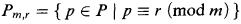Home

# More on an undecidability result of Bateman, Jockusch and Woods

## Extract

Let P be the set of prime numbers. Theorem 1 of  shows that the linear case of Schinzel's Hypothesis (H) implies that multiplication is definable in 〈ω,+,P〉 and therefore that the first-order theory of this structure is undecidable. Let m be any fixed natural number >2, let R be the set of natural numbers <m which are prime to m, and let r be any fixed element of R. The setis infinite (Dirichlet). Theorem 1 of  can be improved as follows:

Proposition. The linear case of Schinzel's Hypothesis (H) implies that multiplication is definable in 〈ω,+,Pm,r〉 and therefore that the first-order theory of this structure is undecidable.

Proof. We follow  with the following new ingredients. Let k be the number of elements of R, i.e. k = ϕ(m) where ϕ is Euler's totient function. Since k is even, the polynomial g(n) = nk + n satisfies g(0) = g(−1) = 0, so (by Lemma 1 of ) it follows from the linear case of (H) that there are natural numbers al (l ϵ ω) such that al+g(0), al+g(1),…, al+g(l) are consecutive primes. Since R is finite, we may assume that all the al's have the same residue t in R, so that al+g(i) ≡ t+1+i (mod m) for i ϵ R. This implies that the function t+1+i (reduced mod m) gives a permutation of R, so we can find s ϵ R such that al+g(s) ≡ r (mod m). Consider the polynomial h(n) = g(s + mn) and let bl = as+ml. Then bl + h(0), bl + h(1),…, bl + h(l) are elements of Pm,r. They are not necessarily consecutive elements of Pm,r, but they are separated by a fixed number of elements of Pm,r. This implies that {h(n) ∣ n ϵ ω} is definable in 〈ω,+,Pm,r〉(by adapting the proof of Theorem 1 of ), and the result follows.

Hide All

# More on an undecidability result of Bateman, Jockusch and Woods

## Metrics

### Full text viewsFull text views reflects the number of PDF downloads, PDFs sent to Google Drive, Dropbox and Kindle and HTML full text views.

Total number of HTML views: 0
Total number of PDF views: 0 *Loading metrics...

### Abstract viewsAbstract views reflect the number of visits to the article landing page.

Total abstract views: 0 *Loading metrics...

* Views captured on Cambridge Core between <date>. This data will be updated every 24 hours.

Usage data cannot currently be displayed.